Function Repository Resource:

# SubTriangle

Construct special triangles of a triangle

Contributed by: Minh Trinh Xuan
 ResourceFunction["SubTriangle"][tri, special] returns the triangle identified by special fom the triangle tri.

## Details

For a triangle ABC, various special triangles are defined:
 AAOA anti-altimedial orthic axes triangle. Ref: X15015 Anticomplementary triangle with ABC as medial triangle. BCI centers of three tangent circles of equal size. Brocard1 coincident points for lines through Brocard points. Brocard2 see references. Brocard3 isogonal conjugate of "Brocard1". Circummedial circumcevian triangle of the centroid. CircumcircleMidArc mid-arc points of the circumcircle. Circumorthic circumcevian triangle of the orthocenter. Contact tangency points of incircle. Euler1 midpoints to the orthocenter. EulerGergonneSoddy right triangle formed by named lines. Excentral centers of excircles, also called the excenters. Extangents triangle tangent to the excircles. Extouch1 tangency points of excircles. Feuerbach 9-point circle tangency points with excircles. HalfAltitude midpoints of altitudes. Incentral cevians of incenter. Intangents the points of pairwise intersection of the three intangents Kosnita the circumcenters of the triangles BOC, COA, AOB Malfatti1 the vertices are the centers of the Malfatti circles Medial midpoints, or cevians of the centroid. Neuberg1 centers of Neuberg circles. Neuberg2 reflections of "Neuberg1". Orthic cevians of orthocenter. Symmedial cevians of symmedian point. Tangential triangle whose incircle is the circumcircle of ABC. Trinh circumcenter reflection of Kosnita triangle. Ref: X7688

## Examples

### Basic Examples (2)

Find and show the anticomplementary triangle:

 In:=Out=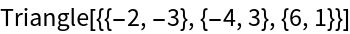In:=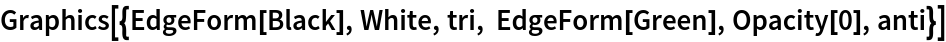Out=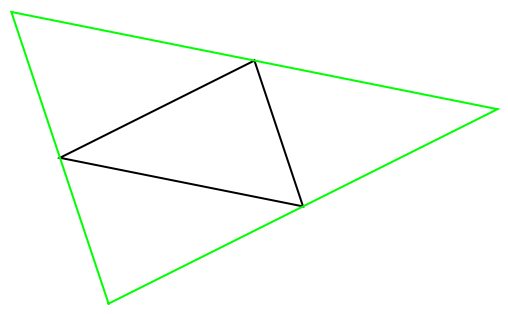Find and show the first Brocard triangle:

 In:=Out=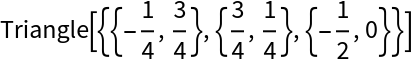In:=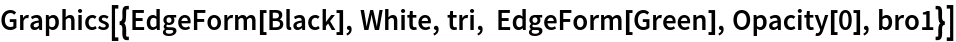Out=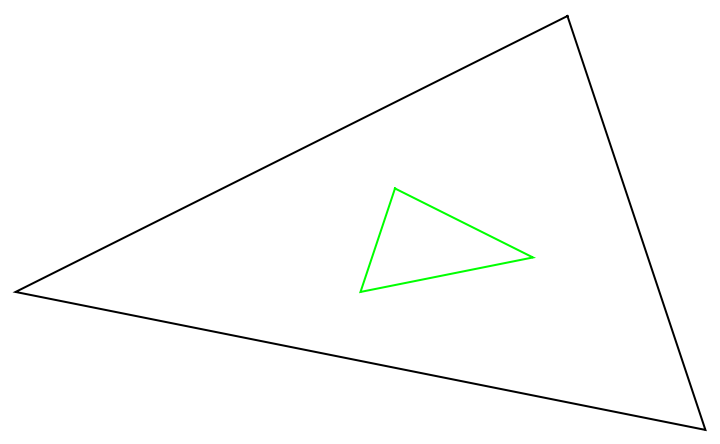### Scope (3)

Find and show the tangential triangle:

 In:=Out=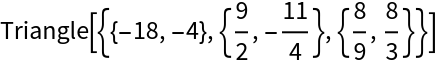The circumcircle of the triangle is the incircle of the tangential triangle:

 In:=Out=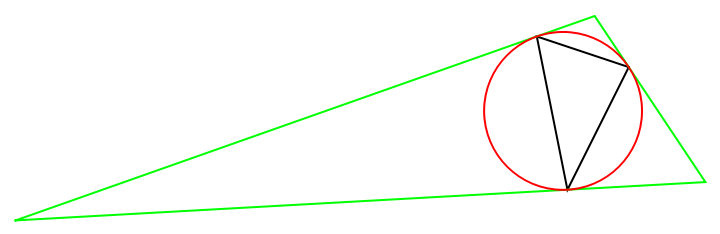Find the half-altitude triangle:

 In:=Out=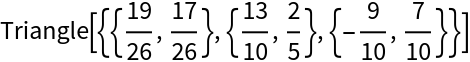The half-altitude triangle is formed by the midpoints of the altitudes:

 In:=Out=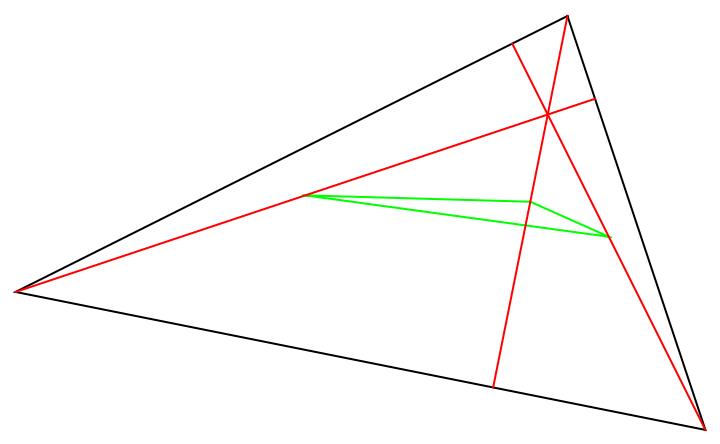Find and show the orthic triangle of the symmedial triangle:

 In:=Out=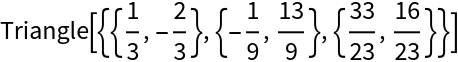In:=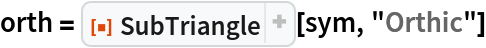Out=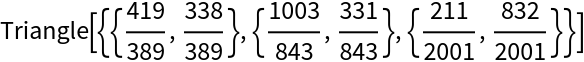In:=Out=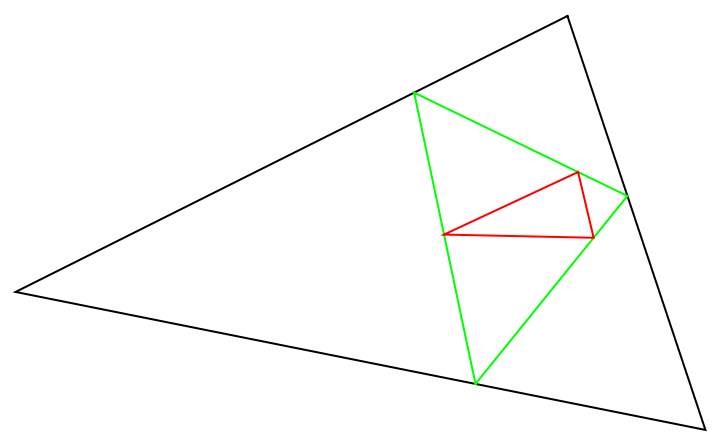### Possible Issues (1)

Special triangles do not always exist:

 In:=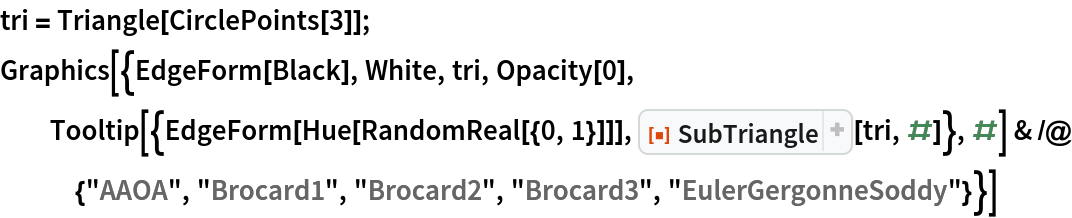Out=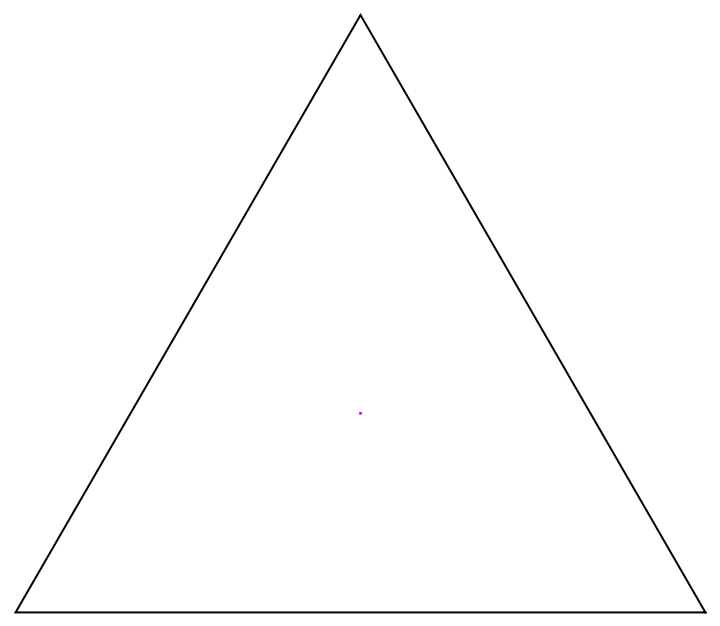### Neat Examples (2)

Show all triangles with Tooltip:

 In:=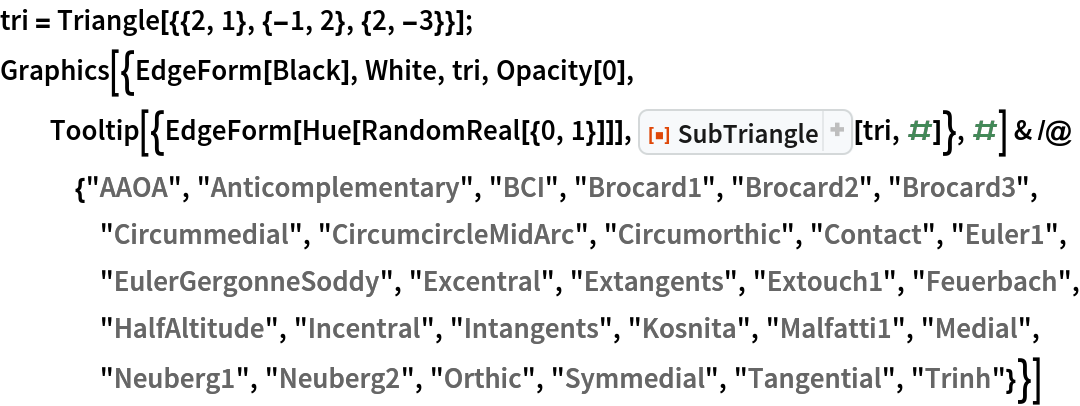Out=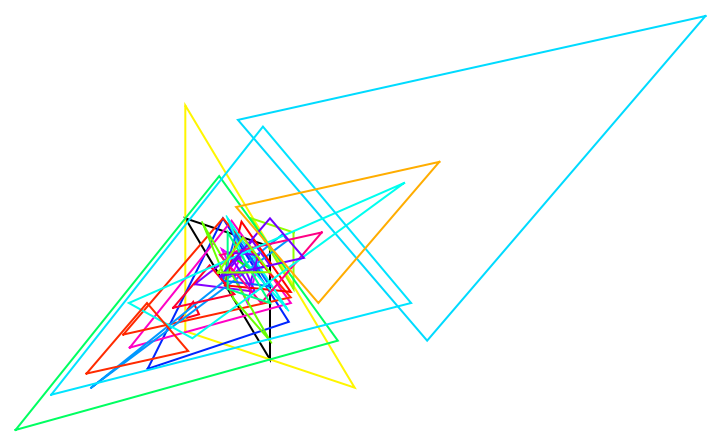Show all triangles in a Manipulate:

 In:=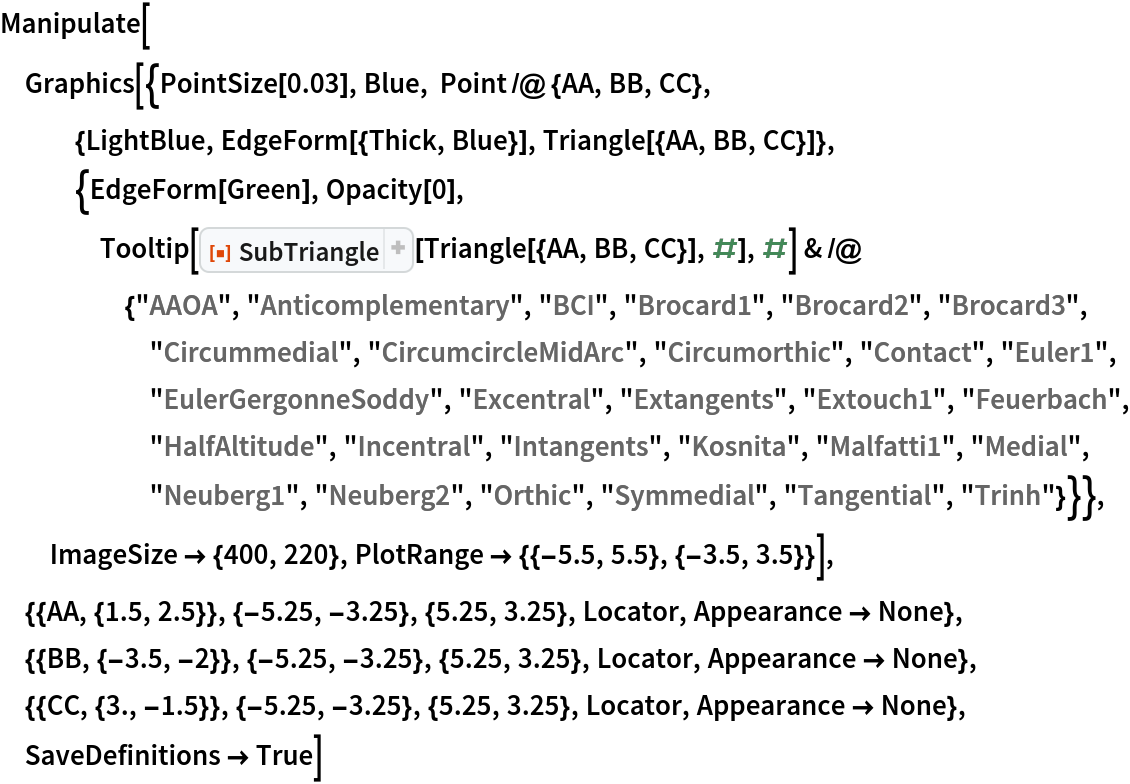Out=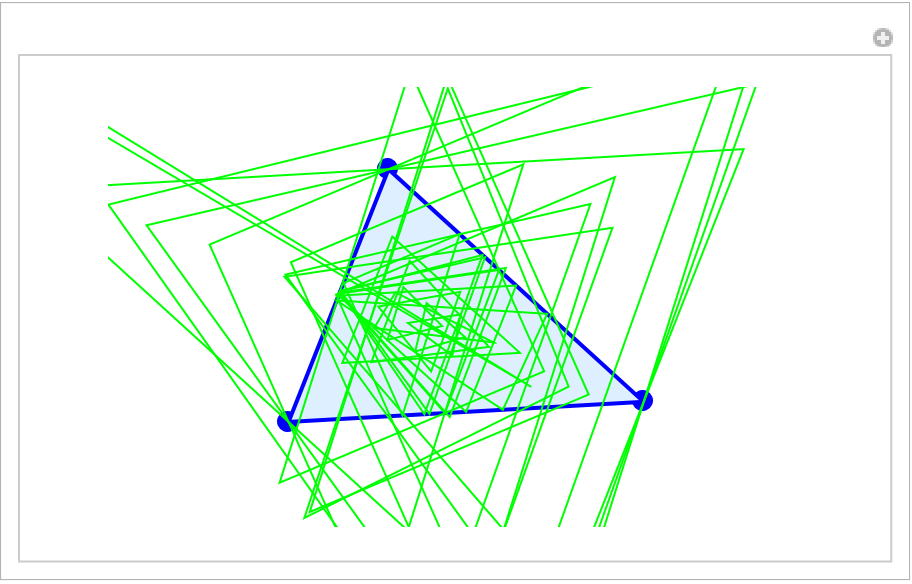Find the Trinh and Kosnita triangles:

 In:=Out=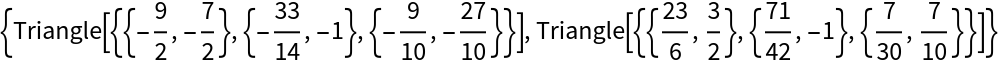The Kosnita and Trinh triangles are symmetric around the circumcenter (in red):

 In:=Out=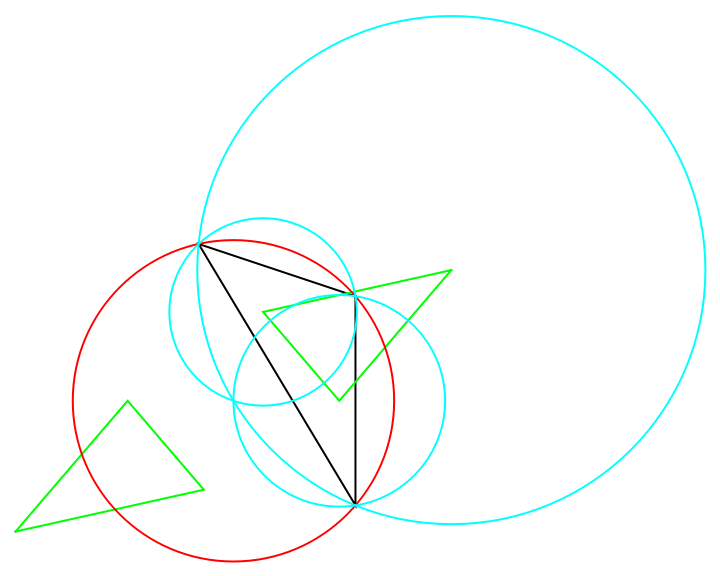## Version History

• 1.0.0 – 07 October 2022